# Data Viz in Julia: Makie¶

I've tried Gadfly and enjoyed the grammar of graphics style of plotting, but I've heard lots of love for Makie as another library worth checking out. I'll use the CairoMakie backend for now, but it looks like the really fun stuff comes with the other backends like GLMakie in terms of interactivity and even making little interact apps.

# Makie¶

## First impressions¶

• The key concepts to think about are:
• Layouts and Figures
• Layoutables
• Plotting Functions
• The Figure object: Plots are created by initializing a Figure object (either explicitly or implicitly with a plotting function call like lines)
• Example: f = Figure(resolution = (600, 400))
• Like the size of the canvas, and you can specify how Layoutables like axes will go
• Layouts
• Makie supports some complex layouts - with your Figure object you can add subplots by adding axes
• Layoutables
• Axes and Legends are examples of Layoutables - what they call stuff that you can add to the Layout.
• Plotting Functions
• i.e. lines, scatter, series, etc.
• Calling a plotting function without referencing a figure will implicitly create a Figure
• What makes Makie so verbose is the fact that there are so many possible attributes that you can modify and set to customize the heck out of your chart.
• See plotting functions documentation for full list of attributes
• Observables
• Special workflow for interactive visualizations
• An Observable is an object that a Listener (usually a function) will react to whenever the Observable changes

Overall, the workflow feels like matplotlib or base plotly in that you're creating a canvas with your Figure and then adding components with Plotting Functions or stuff like Axes and Legends to that Figure incrementally. Each component has a bunch of attributes that you can fiddle around with and tweak to get the customized visualization that you want.

In :
using CairoMakie, ColorSchemes
using DataFramesMeta
using AlgebraOfGraphics, PalmerPenguins

ENV["COLUMNS"] = 1000;

In :
x = range(0, 10, length=100)
y = sin.(x)
lines(x, y)

Out: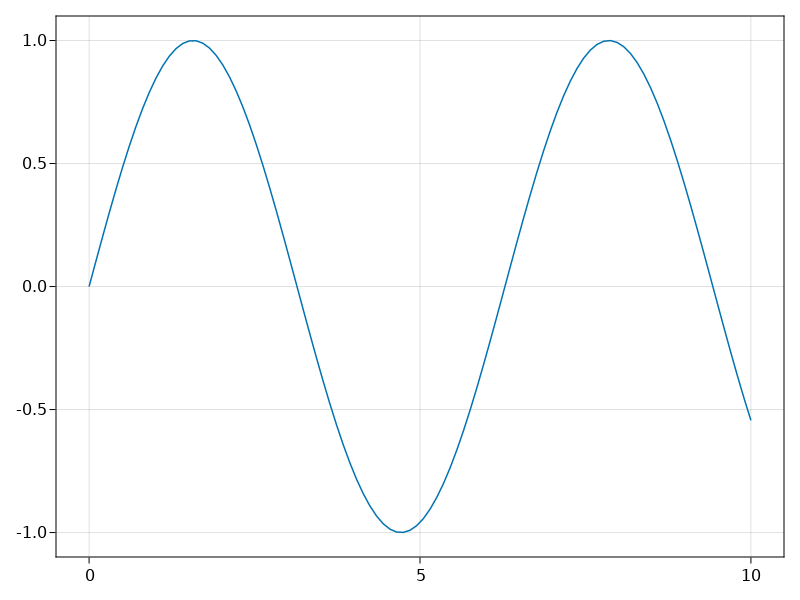The lines call can be unpacked into a figure, axis, and plotobject separately (aka a FigureAxisPlot). Use attributes to see what kinds of atributes you can set on any of these:

In :
f, ax, pltobject = lines(x, y)
pltobject.attributes

Out:
Attributes with 18 entries:
color => RGBA{Float32}(0.0,0.447059,0.698039,1.0)
colormap => viridis
cycle => [:color]
depth_shift => 0.0
diffuse => Float32[0.4, 0.4, 0.4]
fxaa => false
inspectable => true
linestyle => nothing
linewidth => 1.5
model => Float32[1.0 0.0 0.0 0.0; 0.0 1.0 0.0 0.0; 0.0 0.0 1.0 0.0; 0.0 0.0 0.0 1.0]
nan_color => RGBA{Float32}(0.0,0.0,0.0,0.0)
overdraw => false
shininess => 32.0
specular => Float32[0.2, 0.2, 0.2]
ssao => false
transformation => Automatic()
transparency => false
visible => true

Mutating plot functions (i.e. scatter!) allow you to add to an existing figure:

In :
x = range(0, 10, length=100)
y1 = sin.(x)
y2 = cos.(x)

scatter(x, y1, color = :red, markersize = 5, figure=(; resolution=(600, 400)))
scatter!(x, y2, color = :blue, markersize = 10)
current_figure()

Out: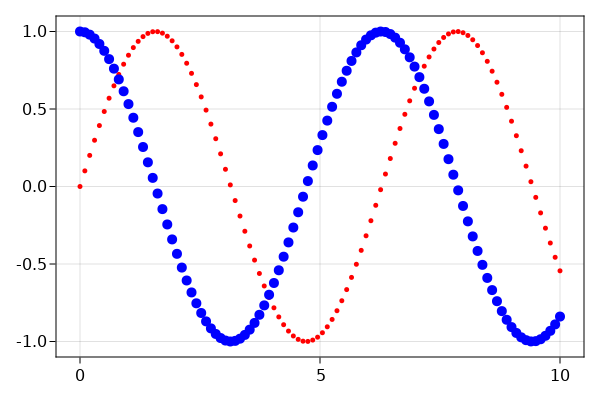In fact, you can specify pretty much anything within the plot creation function:

In :
lines(1:10, (1:10).^2; color=:black, linewidth=2, linestyle=:dash,
figure=(; figure_padding=5, resolution=(600, 400), font="Arial",
backgroundcolor=:grey90, fontsize=16),
axis=(; xlabel=L"x", ylabel=L"x^{2}", title="Chart Title",
xgridstyle=:dash, ygridstyle=:dash))
current_figure()

Out: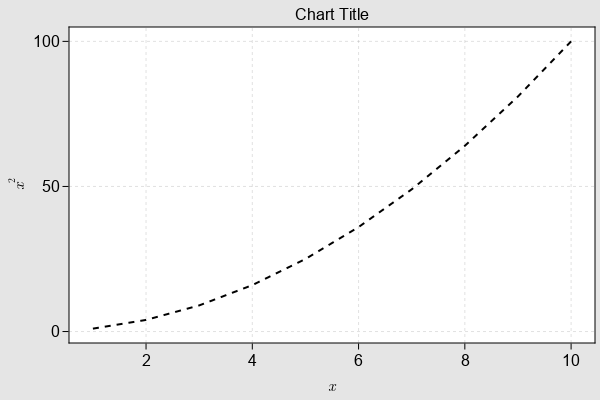Makie can take a matrix object as well, and there's a series plotting function that can do multiple lines at once:

In :
num_lines = 12
dat2 = cumsum(randn(num_lines, 101), dims = 2)
labels=["label $i" for i in 1:num_lines] # See available_gradients() for a list of available colors you can use # Makie will error if you don't have enough colors here fig, ax, sp = series(dat2, labels=labels, color=:tableau_20, figure=(; resolution=(640,400))) axislegend(ax) current_figure()  Out: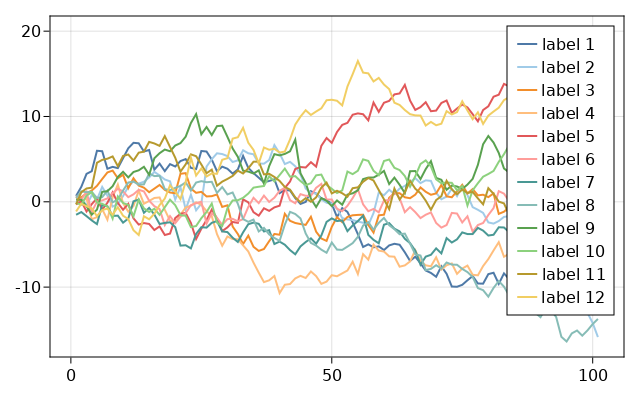## Aside: Color Maps with ColorSchemes¶ One gripe with Makie: you need to specify each color in your lines call, just like in matplotlib. So how do you 'cycle' through a bunch of colors for each of your lines? 1. ColorSchemes.colorschemes[:viridis]: Returns a dictionary of colortypes in the :viridis scheme 2. cgrad(colors, [values]; ): Construct a Colorgradient from colors and values 3. cmap = cgrad(colorschemes[:virids], 20): Creates an array of 20 colors selected from the :viridis color scheme In : num_lines = 12 cbarPal = :viridis cmap = cgrad(colorschemes[cbarPal], num_lines, categorical = false) xs = 0:100 fig = Figure(resolution = (600, 400), font = "CMU Serif") ax = Axis(fig[1, 1], aspect = 1, xlabel = L"x", ylabel = L"x^{p}", xlabelsize = 20, ylabelsize = 20) [lines!(xs, dat2[i, :], color=cmap[i], label="Row$i") for i in 1:num_lines]

Legend(fig[1, 2], ax, nbanks = 2)
display(fig)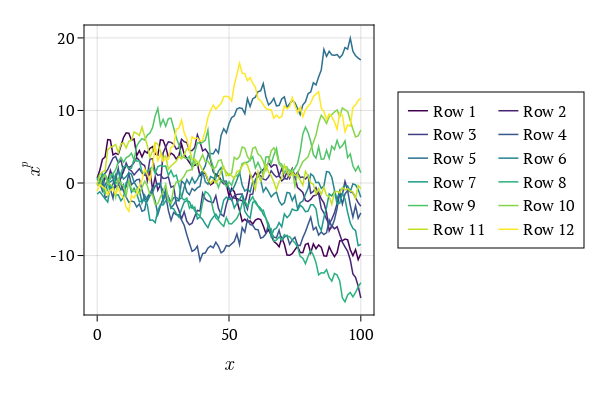Julia for Data Science has a great article here on makie colors: https://juliadatascience.io/makie_colors

Here, we could define a global Theme with a new cycle for colors, however that is not the recommend way to do it. It’s better to define a new theme and use as shown before. Let’s define a new one with a cycle for :color, :linestyle, :marker and a new colormap default. And add these new attributes to our previous publication_theme.

In :
# https://juliadatascience.io/makie_colors

function new_cycle_theme()
# https://nanx.me/ggsci/reference/pal_locuszoom.html
my_colors = ["#D43F3AFF", "#EEA236FF", "#5CB85CFF", "#46B8DAFF",
"#357EBDFF", "#9632B8FF", "#B8B8B8FF"]
cycle = Cycle([:color, :linestyle, :marker], covary=true) # alltogether
my_markers = [:circle, :rect, :utriangle, :dtriangle, :diamond,
:pentagon, :cross, :xcross]
my_linestyle = [nothing, :dash, :dot, :dashdot, :dashdotdot]
Theme(
fontsize=16, font="CMU Serif",
colormap=:linear_bmy_10_95_c78_n256,
palette=(color=my_colors, marker=my_markers, linestyle=my_linestyle),
Lines=(cycle=cycle,), Scatter=(cycle=cycle,),
Axis=(xlabelsize=20, xgridstyle=:dash, ygridstyle=:dash,
xtickalign=1, ytickalign=1, yticksize=10, xticksize=10,
Legend=(framecolor=(:black, 0.5), bgcolor=(:white, 0.5)),
Colorbar=(ticksize=16, tickalign=1, spinewidth=0.5),
)
end

function scatters_and_lines()
x = collect(0:10)
xh = LinRange(4, 6, 25)
yh = LinRange(70, 95, 25)
h = randn(25, 25)
fig = Figure(resolution=(600, 400), font="CMU Serif")
ax = Axis(fig[1, 1], xlabel=L"x", ylabel=L"f(x,a)")
for i in x
lines!(ax, x, i .* x; label=L"$(i) x") scatter!(ax, x, i .* x; markersize=13, strokewidth=0.25, label=L"$(i) x")
end
hm = heatmap!(xh, yh, h)
axislegend(L"f(x)"; merge=true, position=:lt, nbanks=2, labelsize=14)
Colorbar(fig[1, 2], hm, label="new default colormap")
limits!(ax, -0.5, 10.5, -5, 105)
colgap!(fig.layout, 5)
fig
end

with_theme(scatters_and_lines, new_cycle_theme())

Out: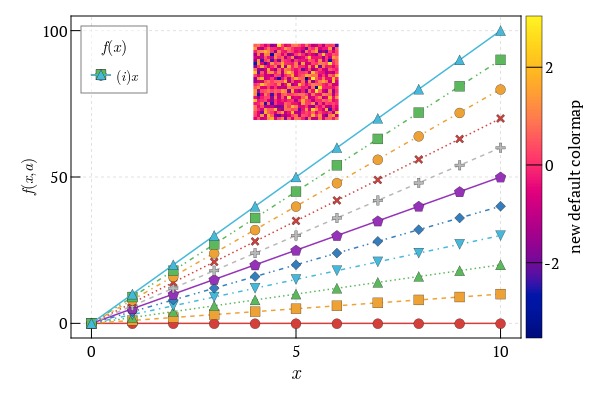We can see that Makie works just fine for vectors and matrices, but what about DataFrames? Well, each column of a dataframe is a vector so you could get by with loops, but we have to write a lot of extra throwaway code which can get annoying fast.

# AlgebraOfGraphics¶

While Makie is powerful, it does seem a little verbose if you work with tabular data in DataFrames. It's recommended if you want to build complex or 'publication quality' plots or you want interactivity, but it could use a wrapper to make things faster for one-off plots.

ggplot2 is still the go-to plotting library for R, and using it within the tidyverse feels like a well thought out, integrated system that is both easy to use and easy to layer complexity on top of it.

Gadfly follows very closely in the ggplot2 Grammar of Graphics style, so it already feels familiar (dare I say pleasing) to use with DataFramesMeta and comes with plenty of customization right out of the bat.

What makes the GoG-style plotting libraries feel so powerful is that you can add or remove layers of complexity on top of the plot without having to rewrite a lot of code. It's flexible in that the core building blocks work together seamlessly once you learn the grammar.

Enter AlgebraOfGraphics. AlgebraOfGraphics uses Makie in the background so that you still have all of the options with layouts and attributes, but it allows you to write similarly expressive statements that give you some of the benefits of GoG. However, it's just different enough that it takes some getting used to.

We can start with an example and you can see the code in action:

In :
# on the first run, if it asks for stdin type in 'y' and hit enter to download
first(penguins, 6)

Out:

6 rows × 7 columns (omitted printing of 1 columns)

speciesislandbill_length_mmbill_depth_mmflipper_length_mmbody_mass_g
String15String15Float64Float64Int64Int64
In :
set_aog_theme!() # just like seaborn's sns.set()

axis = (width = 225, height = 225)
penguin_frequency = data(penguins) * frequency() * mapping(:species)

draw(penguin_frequency; axis)

Out: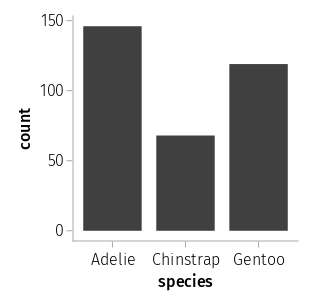The line:

penguin_frequency = data(penguins) * frequency() * mapping(:species)


is an example of the Algebra of Layers, where Layers are the key building block or abstraction. Each layer is a product of a combination of elementary objects:

• Data
• Mapping: associate data to plot attributes
• Visual: plotting functions from Makie
• Analyses: i.e. Histogram, frequency table

And finally, once you've specified the Layers you use the draw function to render it:

draw(penguin_frequency) # renders the Layers


Let's revisit our first lines plot from Makie using this AoG language:

In :
df = DataFrame(x = range(0, 10, length=100), y = sin.(x))
plt = data(df) * mapping(:x, :y)
draw(plt * visual(Lines); axis)

Out: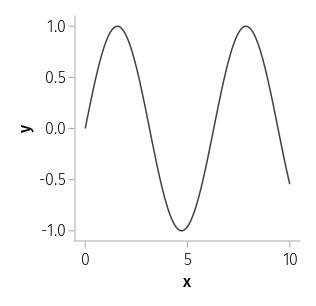The multiplication operator (*) is being used to add objects within the same layer, while the addition operator (+) is used to combine more than one Layer into a list of Layers.

The draw function operates on either Layer or Layers and assigns it to the specified figure or axis

In :
df = DataFrame(dat, :auto)
x2 = data(df) * mapping(:x1, :x2) * visual(Lines, color="orange")
x3 = data(df) * mapping(:x1, :x3) * visual(Lines, color="green", linewidth=3)
# Use the addition operator to combine our two Layer(s)
draw(x2 + x3; axis)

Out: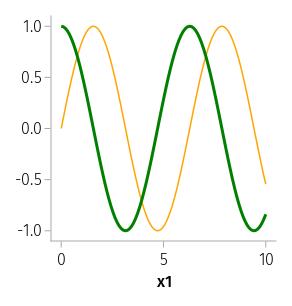It's still a little early for AlgebraOfGraphics but it's nice to see the GoG style on top of the power of Makie. I'd still prefer to use Makie itself or Gadfly for one-off plots - and knowing a lower level library is always useful.

In [ ]: